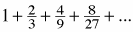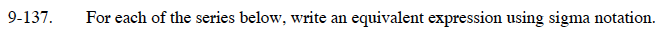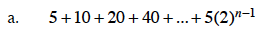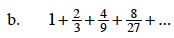### Home > CALC > Chapter 9 > Lesson 9.4.3 > Problem9-137

9-137.
1. For each of the series below, write an equivalent expression using sigma notation. Homework Help ✎

1. 5 + 10 + 20 + 40 + ... + 5(2) n- 1

2.$\sum_{i=1}^{n}5(2)^{i-1}$$a_n=\Big(\frac{2}{3}\Big)^n\text{ starting with }n=0.$